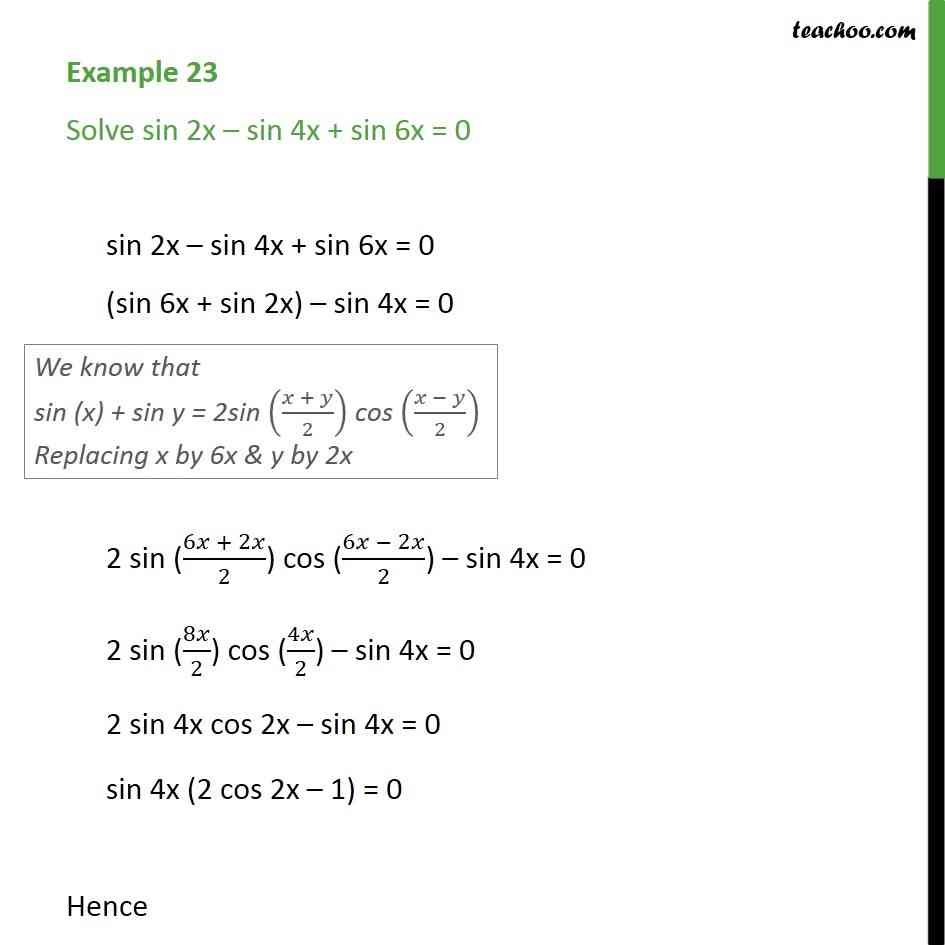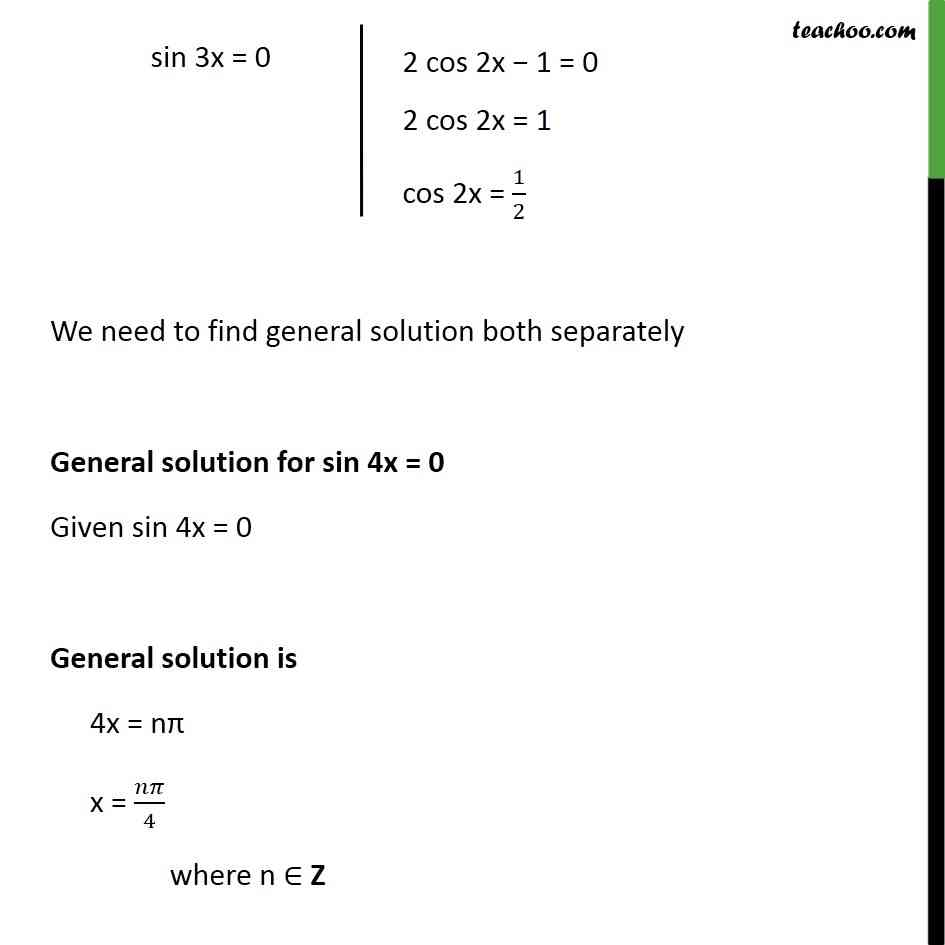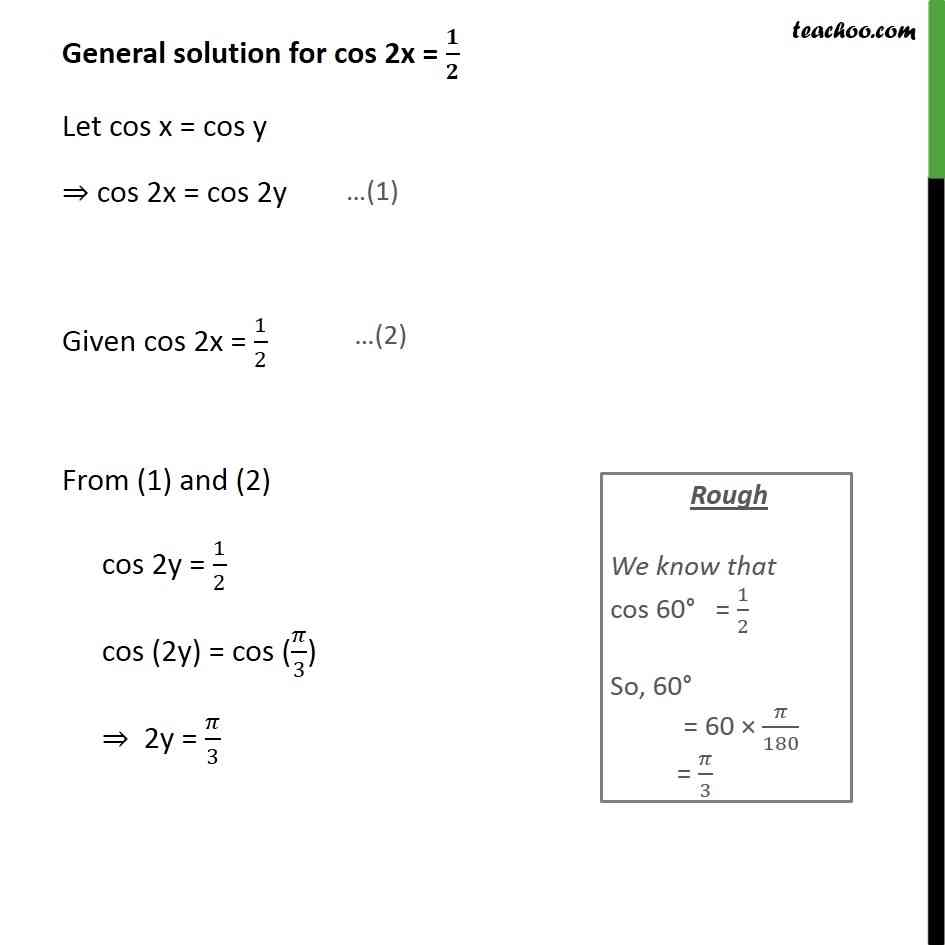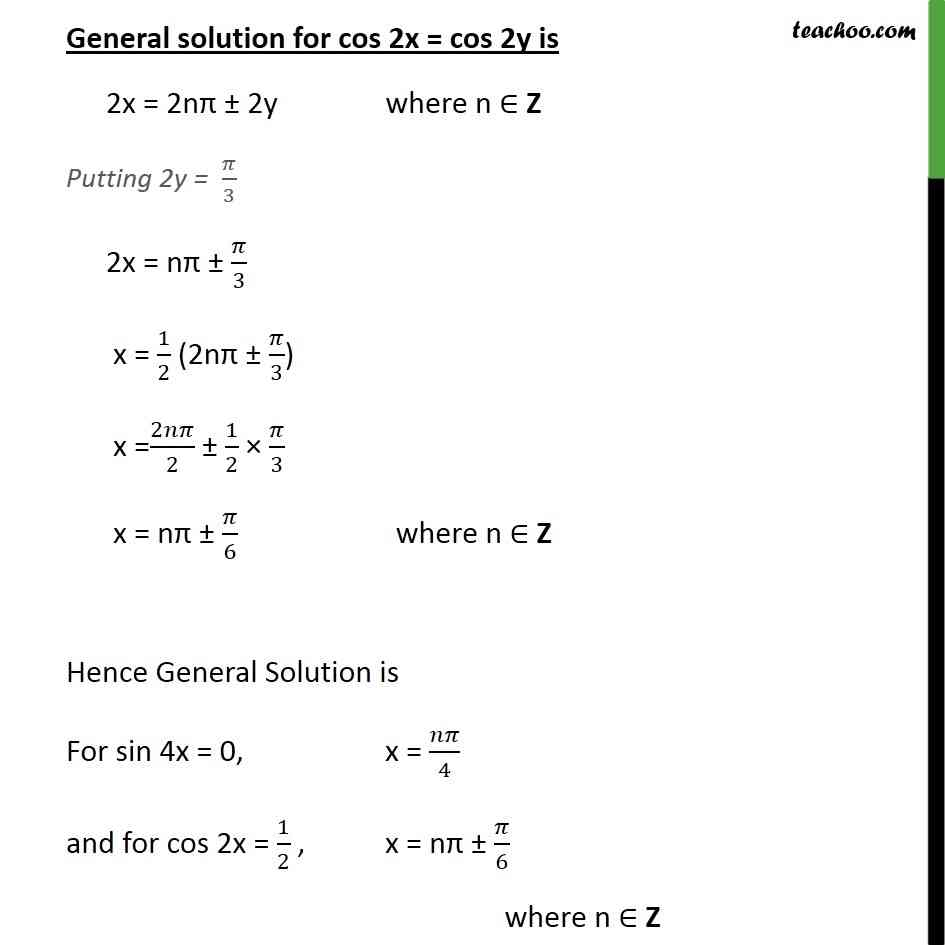Finding General Solutions

Chapter 3 Class 11 Trigonometric Functions
Concept wiseLearn in your speed, with individual attention - Teachoo Maths 1-on-1 Class

### Transcript

Question 6 Solve sin 2x sin 4x + sin 6x = 0. sin 2x sin 4x + sin 6x = 0 (sin 6x + sin 2x) sin 4x = 0 2 sin ((6 + 2 )/2) cos ((6 2 )/2) sin 4x = 0 2 sin (8 /2) cos (4 /2) sin 4x = 0 2 sin 4x cos (2x) sin 4x = 0 sin 4x (2 cos (2x) 1) = 0 Hence sin 4x = 0 or 2cos 2x 1 = 0 sin 4x = 0 or 2cos 2x = 1 sin 4x = 0 or cos 2x = 1/2 We need to find general solution both separately General solution for sin 4x = 0 Let sin x = sin y sin 4x = sin 4y Given sin 4x = 0 From (1) and (2) sin 4y = 0 sin 4y = sin (0) 4y = 0 y = 0 General solution for sin 4x = sin 4y is 4x = n (-1)n 4y where n Z Put y = 0 4x = n (-1)n 0 4x = n x = /4 where n Z General solution for cos 2x = / Let cos x = cos y cos 2x = cos 2y Given cos 2x = 1/2 From (3) and (4) cos 2y = 1/2 cos (2y) = cos ( /3) 2y = /3 General solution for cos 2x = cos 2y is 2x = 2n 2y where n Z putting 2y = /3 2x = n /3 x = 1/2 (2n /3) x =2 /2 1/2 /3 x = n /6 where n Z Hence General Solution is For sin4x = 0, x = /4 and for cos 2x = 1/2 , x = n /6 where n Z